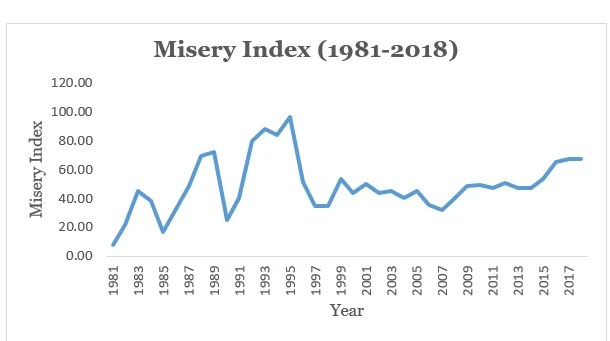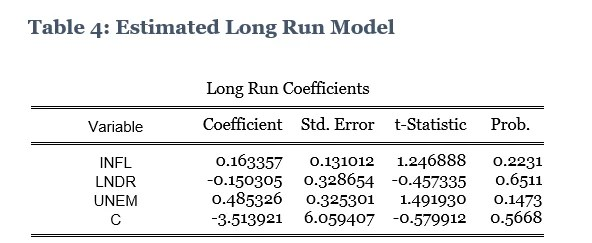The misery index is a measure of how miserable the citizens of a country can be at a particular period of time. It is measured by evaluating the addition of Inflation Rate, Unemployment Rate and Lending rate, minus Year-On-Year growth in GDP per Capita. This shows that the higher the misery index, the more the citizens are in a miserable state and the lower the misery index, the higher the level of happiness in the country. Alternatively, it can be said that a miserable state is associated with lower level of employment, higher lending rates, higher inflation rate and a lower growth in GDP per Capita.

Based on the data gotten 2018 CBN statistical bulletin, Nigerian Bureau of Statistics, IMF World Economic Outlook Data Base and Index Mundi, this study seeks to examine the misery index level of Nigeria from 1981 to 2018 while determining the relationship that exists among the different components that constitute the misery index.

Chart 1: Nigeria’s Misery Index (1981-2018)Source: IMF, NBS, CBN, Gifted Hands Research.

Aided by 28% increase in the growth of income per capita level in 1981, the misery index recorded lowest at an index level of 7.53% and this shows that about 92% of the citizens are happy. However, this index level increased at an increasing rate from 1982 to 1984 until it increased at a decreasing rate in 1985. The year 1995 saw the misery index at its highest point of 96.43% thanks for the record level of the inflation rate at 72.84%, but this was short lived as it dropped sharply in 1996 and 1997. In 2018, the misery index level of Nigeria was down by 0.49 as the country recorded a misery index value of 67.09 down from 67.58 recorded in the previous year (2017).

Chart 2: Relationship between Inflation and Unemployment Rate (1981-2018)Source: NBS, CBN, Gifted Hands Research.

The chart above shows that up until 1987, there was a positive relationship between inflation rate and the rate of unemployment. In 1981 when the inflation rate was 20.81%, unemployment rate stood at 5.20%. As this rate reduced to 7.7% in 1982, the unemployment rate also fell to 4.30% in the same period. This relationship lasted till 1987 and we can denote this as the short run.

However, from 1988 to date, the trend has been such that as the rate of inflation increases, the rate of unemployment decreases and vice versa. This falls in line with the observation of A.W Phillips through his famous Phillips curve which shows the inverse relationship between inflation and unemployment. That is, if unemployment is high, inflation will be low and if unemployment is low, inflation will be high.

PRESENTATION OF ESTIMATED MODEL(S)

The test for unit root using ADF test was conducted in order to ascertain whether time series data were stationary or non-stationary and also to determine the number of times (the level) at which the variables have to be differenced before becoming stationary.Source: Author’s Computation, 2019 (E-views 9).

The Mackinnon values give the critical values for the determination of the order of integration. The values of the Mackinnon and the ADF test statistics are compared and decisions either to reject or accept the null hypothesis are taken. By taking the values of each variable in absolute terms, it will be observed that not all the variables are stationary at level as some are stationary at first difference and hence, Lending rate and unemployment are regarded as I (1) series while inflation rate and GDP per capita are regarded as I (0) series. Also, they are all significant at 5%.

Since the variables are combinations of I (0) and I (1) series, the ARDL bound test is the most suitable for ascertaining whether or not a long run relationship exist among the variables.

Co-integration / long-run relationship test

The ARDL bound test will be carried out to ascertain whether a long run relationship really exists among the variables that have been observed to be stationary at level and first difference. The ARDL bound test result will be interpreted by comparing the F-Statistics value with the critical values (lower and upper bound) and the decision will be based on the null hypothesis which states: No long-run relationships exist. If the F-Statistics value is less than the lower bound value (I0 bound) at 5% significance, then do not reject the null hypothesis and conclude that no long run relationship exist. However, if the F-Statistics value is greater than the upper bound value (I1 bound) at 5% significance level, then there exist a long run relationship. But if the F-Statistics falls in-between the lower bound and upper bound values at 5% significance level, then the result is inconclusive.

The ARDL bound test result is summarized below:Source: Author’s Computation using E-views 9.0 (2019)

From the results above, the F-Statistics value of 5.027070 exceeds the upper bound (I1 Bound) value of 4.35 at 5% significance level resulting to the rejection of the null hypothesis. The conclusion therefore is that there exists a long run relationship among the variables.

Since the ARDL bound test result revealed that a long run relationship exists among the variables, the ARDL co-integrating and long run form which is a short run Error Correction technique will be carried out to ascertain the short run and long run model.Source: Author’s Computation using E-views 9.0 (2019)

The coefficient of CointEq (-1) represents the Error Correction Model (ECM) term which must be negative and significant and this represents the speed of adjustment from short run equilibrium to long run equilibrium. The above CointEq (-1) coefficients signifies that 69% error is corrected from short run to the long run for each time period.

The short run model result shows that the coefficient of D (INFL) is about 0.013 which implies that a one percentage increase in inflation rate increases the GDP per capita by 0.013%. Similarly, the coefficient of D(LNDR) is 0.295 and this denotes that a percentage increase in lending rates in the short run, leads to 0.029% growth in YOY GDP per capita. Furthermore, the coefficient of D(LNDR(-1)) shows that a percentage increase in lending rates with a one year lag will increase YOY growth rate in GDP per capita by 0.42%. Lastly, the coefficient of D(UNEM) shows that an increase in the rate of unemployment will bring about a decrease in growth rate of GDP per capita by 0.23%.

It is however noteworthy that in the short run, all these variables are not statistically significant in influencing YOY growth rate in GDP per capita as their probability values are all greater than the chosen level of significance (5%).Source: Author’s Computation using E-views 9.0 (2019)

The above ARDL long run form result also shows that although there are relationships among the various components of the misery index, they are not all significant in determining and influencing the yearly growth rate in GDP per capital. The table shows that in the long run, a percentage increase in the rate of inflation will lead to 0.16% increase in YOY growth rate in GDP per capita. Also, the coefficient of lending rate is -0.150 which denotes that an increase in lending rates in the long run will lead to a decrease in YOY growth in GDP per capita by 0.15%. Also, a percentage increase in unemployment rate will lead to 0.48% increase in yearly growth in GDP per capita in the long run.

Residual Diagnostic Test

Various residual diagnostics tests were carried out to assess the validity of the model. These include: testing if the residuals are normally distributed, serially correlated, presence of heteroskedasticy and testing for specification errors. The result for these tests are presented in table 5 below.Source: Author’s Computation using E-views 9.0 (2019)

To test if the residuals are normally distributed or not, the Jarque –Bera Normality Test was conducted and the result showed a P-value of 0.829861 which is greater than 5% level of significance thereby implying that the model is normally distributed. The Breush_Godfrey Serial Correlation LM Test results has a P-value of 0.7646 which also is greater than 5% thereby signifying that the residuals are not serially correlated and likewise the Breush-Godfrey Hetereskodasticity reveals an absence of hetereskodasticity in the model given its associated p-value of 0.1460 which is greater than 5%. The Ramsey Test showed evidence that the functional form of the model is properly specified given an associated P-value of 0.3561 which is greater than 5% significance level.

In summary, the residual diagnostic tests result reveals that the residual is not serially correlated, absence of heteroskedasticity, absence of specification error and that the model is normally distributed. Since the error term is well-behaved, inferences made from the estimated model are valid.

CONCLUSION

From the foregoing, it can be seen that there is a trade-off between inflation and unemployment and this follows the Philips curve. However, this is not necessarily true in the short run as a higher inflation rate leads to higher level of unemployment. While making YOY growth in GDP per capita as the dependent variable, the study also showed the relationship that exists among the different components of misery index (Inflation rate, lending rate, and unemployment rate) although these relationships are not statistically significant.

Therefore, the results showed that inflation has a positive effect on YOY growth in GDP per capita both in the long run and in the short run. However, while lending rate has a positive relationship with YOY growth in GDP per capita in the short run, this relationship is negative in the long run. Lastly, the study also established that there is a negative between unemployment and YOY growth in GDP per capita in the short run but a positive relationship between the two variables in the long run.

RELATED ARTICLES